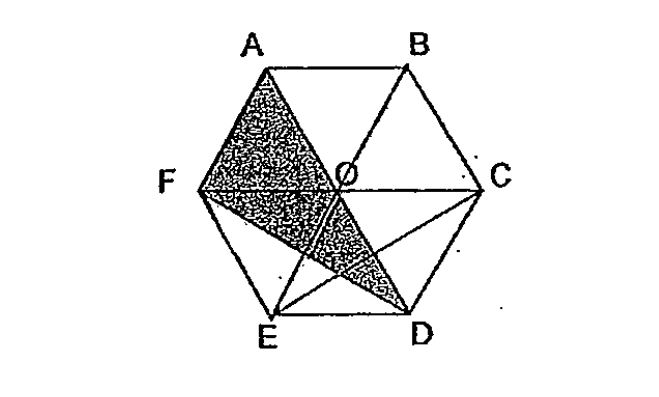# Question 1 of 31

In the diagram below, ABCO and FODE are identical rhombuses and AOF and OCD are identical equilateral triangle. What fraction of the figure ABCDEF is shaded?A
$\frac{1}{2}$
B
$\frac{1}{3}$
C
$\frac{1}{4}$
D
$\frac{1}{5}$
E
None of the above